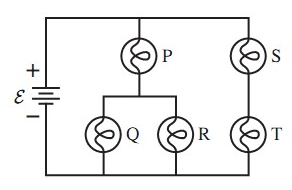# Problem: The five identical bulbs in the following figure are all glowing. The battery is idealWhat is the order of brightness of the bulbs, from brightest to dimmest? Some may be equal.

###### FREE Expert Solution

Equivalent resistance for series resistors:

$\overline{){{\mathbf{R}}}_{\mathbf{e}\mathbf{q}}{\mathbf{=}}{{\mathbf{R}}}_{{\mathbf{1}}}{\mathbf{+}}{{\mathbf{R}}}_{{\mathbf{2}}}}$

Equivalent resistance for parallel resistors:

$\overline{)\frac{\mathbf{1}}{{\mathbf{R}}_{\mathbf{eq}}}{\mathbf{=}}\frac{\mathbf{1}}{{\mathbf{R}}_{\mathbf{1}}}{\mathbf{+}}\frac{\mathbf{1}}{{\mathbf{R}}_{\mathbf{2}}}}$

Ohm's law:

$\overline{){\mathbf{V}}{\mathbf{=}}{\mathbf{iR}}}$

Connecting two resistors with equal resistance in parallel halves their resistance.

Connecting two resistors with equal resistance in series doubles their resistance.

The resistance of the branch ST is equal to 2R.

98% (494 ratings)###### Problem Details

The five identical bulbs in the following figure are all glowing. The battery is idealWhat is the order of brightness of the bulbs, from brightest to dimmest? Some may be equal.